## The hardest sudokus (new thread)

Everything about Sudoku that doesn't fit in one of the other sections

### Re: The hardest sudokus (new thread)

Pupp wrote:Thanks. Just curious, but does anybody know the highest difficulty for normal sudoku puzzles they use in competition?

There has to be some sort of upper bound, since solvers have a time limit to solve a puzzle.

the thing about speed solving sudoku puzzles is that once you get beyond a certain difficulty level it is much faster to guess-and-check and rub out your numbers if you guessed wrong. the general consensus in the puzzle solving world is that this point happens at pairs/triples and x-wings and maybe swordfish, which would be a maximum se rating of 4.0. some people use uniqueness too, which would move it up to the 4.5 range

puzzles above this, such as the puzzles in the puzzles section of this forum which are in the 7 range, would not typically be considered suitable for speed solving. if you were to speed solve those you would always either guess and hope you got it right, or do a bifurcation from a certain point until you get a contradiction but not in the way the people posting here use wings or als's or aic's with their fancy notation. they would not consider our solutions to those puzzles here as "beautiful" in the same way we do. beyond this, puzzles in the 8 range would not even be considered as valid sudokus at all

i have a friend who is a speed solver and i had a facebook conversation with him on this topic a couple of years ago and this is what he said
There are some (notably Thomas Snyder aka motris) who believe that locked sets and X wings are the limit. Some people agree with him in principle but not about where the line is.

Some people believe that bifurcation is a valid strategy and that contests should have puzzles that test your ability to choose a useful guessing point and find contradictions.

These camps disagree vehemently. Thomas Snyder boycotted competitions for years because the other camp was winning. He's come back now that most people are on his side (having so many novel variants made it easier to stick to simpler techniques, but some variants obviously make certain techniques easy(ier) that are normally difficult in standard puzzles and some have their own set and so on...)

But there are still a few people (mostly central Europeans like the Czechs the Slovaks and the Slovenes) who like guessing puzzles

So it pays to know who's who when solving because the author might be in the guessing camp

(thomas snyder was the world champion for a few years i think)

also for some reason the speed solving community absolutely hate platinum blonde (se 10.6? I don't remember exactly) and use that puzzle as an example to poke fun at "purist" sudoku solving communities, like this one, who value hard puzzles for their own sake. I have no idea why they picked platinum blonde and neither does my friend, but he says there is a frequently quoted line that goes "I'd rather do a pretty (insert variant) than platinum blonde" with the underlying idea that people who like doing puzzles at this difficulty level are delusional and wasting their time. this particular puzzle has become a bit of a meme

maybe strmckr could shed some more light on this topic

edit:
tarek wrote:I’m not sure where to find it now but somebody has a thread with puzzles having the maximal number of clues for each SE rating which is impressive

Tarek

I'm just a "somebody"?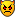alright from now on I'll refer to you as "somebody" and see how you like it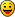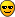999_Springs

Posts: 585
Joined: 27 January 2007
Location: In the toilet, flushing down springs, one by one.

### Re: The hardest sudokus (new thread)

999_springs wrote:maybe strmckr could shed some more light on this topic

i believe strmckr got banned from on line competitions because he was so good .... i believe he used double propositon pairs to solve difficult puzzles very quickly ...
and poor old Thomas Snyder [motris] was rightly pretty miffed when he got pipped at the post [ by a person who guessed] on the last puzzle in the first world championship - despite leading in the previous 9 puzzles ...sigh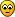but he did make up for it and won the next world championship ...999_springs wrote:
this particular puzzle has become a bit of a meme
...woohoo ... hats off to the platinum blonde ... i didnt know that !!!!coloin

Posts: 2219
Joined: 05 May 2005
Location: Devon

### Re: The hardest sudokus (new thread)

999_Springs wrote:
tarek wrote:I’m not sure where to find it now but somebody has a thread with puzzles having the maximal number of clues for each SE rating which is impressive

Tarek

I'm just a "somebody"?alright from now on I'll refer to you as "somebody" and see how you like itSurly you knew I only said it knowing that you would read itI was actually expecting a quicker response from you!

coloin wrote:
999_springs wrote:
this particular puzzle has become a bit of a meme
...woohoo ... hats off to the platinum blonde ... i didnt know that !!!!It broke the q2 record when it was posted so everybody's within their rights to be cautious about this femme fatale.tarek

Posts: 3759
Joined: 05 January 2006

### Re: The hardest sudokus (new thread)

coloin wrote:these ones posted by ssxsssxs which also are not [yet] in database
Code: Select all
`12.3.....6...7..1...4..5...3.....4...6..2..3...8.....9...9....8.7..3..6......25..  ED=11.7/1.2/1.212.3.....34..1......5..6...2.....5...1.....2...7..8..9...5..6.7....3..4......79..  ED=11.7/11.7/2.6`

I finally got around to adding these to my database; the second one yields another 11.7 by swapping the 5 and 6 in row 3. In minlex form:

Code: Select all
`.....1..2....3..4....5..1.6..1..72...8......59......8...6..5...43..9....89.3.....  ED=11.7/11.7/2.6 (DCFC+DFC) q2=95459`
mith

Posts: 859
Joined: 14 July 2020

### Re: The hardest sudokus (new thread)

Updated the spreadsheet (link). Updating lists of (ER>=11.6 or D*FC+DFC or Q1>=99000 or Q2>=99100):

Code: Select all
`21C........1.....2.3...4.5.6.7...2.8....7..6...59.........16...4...5..1.7..8..3.....  ED=11.7/11.7/2.6  (DCFC+DFC) q1=95705 q2=97905........1.......2...3..45......1..6....7.38...57..8....458..3...7.......6...9...2  ED=11.6/11.6/2.6  (DCFC+DFC) q1=95076 q2=98167........1.....234...1.5........6...7...8...3..4...98...8.2...9.1........5.6.7...4  ED=11.2/11.2/9.2  (DCFC+MFC) q1=99244 q2=98766.................1..2.34.5...36.7....2.8.....5...2..7...5.......3..52.4.7.4...9..  ED=10.9/1.2/1.2   (DLFC+MFC) q1=99218 q2=98850........1.....2.3...4.5.6.7...2.8....7..6.1..9.........16.....4.5..1.7..8..3.....  ED=11.4/1.2/1.2   (DCFC+MFC) q1=99131 q2=98848........1.....2.3...3.4.5.....6.......1.7...4.8...97....5.1..7..6...8...9..2..4..  ED=10.8/1.2/1.2   (DCFC+FC)  q1=99081 q2=98868........1.....2..3..4.5..6...5....7..3....8..7..94......65...9..1...8....2.67....  ED=10.6/10.5/2.6  (DCFC+FC)  q1=99071 q2=98769........1..2..3.4..4..5.6.......4.7...41....38...9......17....2.5..6....9.....8..  ED=11.5/11.5/10.0 (DCFC+MFC) q1=97523 q2=9940022C........1.....2.3...4.5.6...3...1...4.78.....6..54.....4....7...9.....127...6.8..  ED=11.8/1.5/1.5   (DCFC+DFC) q1=98819 q2=97704........1..2..3.4..4..5.6.......7.8...43......1..9.5...9......63.78.....4....2.7.  ED=11.7/11.7/10.7 (DCFC+DFC) q1=95075 q2=97539........1.....2.3...4.5.6.....2...7..7...4..28...9.5....5..69...1.3.....6.8.4....  ED=11.7/11.7/9.4  (DCFC+DFC) q1=97299 q2=97638........1.....2.34..3.5.6.....6..7...7..8....9....1.4..8.5.....3.1....9.4....3..2  ED=11.7/11.7/8.0  (DLFC+DFC) q1=95031 q2=97177.....1..2....3..4....5..1.6..1..72...8......59......8...6..5...43..9....89.3.....  ED=11.7/11.7/2.6  (DCFC+DFC) q1=95459 q2=98416........1.....1..2...34..5...36......574.....8....2....46....7..7.5...6.9...6.1..  ED=11.7/11.7/2.6  (DCFC+DFC) q1=95012 q2=95198........1.....2.....3.4.56....7.......4.......5..3.42..26.....8.35..9...4...2.35.  ED=11.7/1.2/1.2   (DLFC+DFC) q1=95807 q2=98760........1....23.4.....452....1.....6.3...6.2.6.7...8....8.5.....5...4...1..6....9  ED=11.7/1.2/1.2   (DRFC+DFC) q1=75392 q2=95978........1.....2.3.....4.5.6..6.5...4.51...7..8..2......6.8...9..7..1.6..9....3...  ED=11.6/11.6/8.0  (DCFC+DFC) q1=95049 q2=98089........1.....2.....3.4..5........6...7...4.3.6..3.7.5.35.6..7.8...5..4.9..8.....  ED=11.4/1.2/1.2   (DLFC+DFC) q1=95813 q2=98927..............1.23..4.5.6.......2.7...8.4.5...7.3....1..79....5.6..7....84....7..  ED=10.1/1.2/1.2   (DCFC+)    q1=99412 q2=99403........1.....2.34..5.6.2......7.....6...43..8.29.......78...2.23...61..9........  ED=10.5/1.2/1.2   (DLFC+FC)  q1=99386 q2=99391........1....23.4...56..7.......4.....85..6...2..1..3...2.9...675....2..8..2.....  ED=10.5/1.2/1.2   (DLFC+FC)  q1=99386 q2=99391........1..2..3.4..5..6.7.......1.23.7..8.6..2..4........6...9.5.....2..8.7.2....  ED=10.5/1.2/1.2   (DLFC+FC)  q1=99386 q2=99391........1..2..3.4..4..5.6.....6.......7..8.2..9..1...5..98..4...7.....9.32...9...  ED=10.4/10.4/9.6  (DRFC+FC)  q1=99381 q2=99375........1.......23..2..34......5.6....6..1..4.7.8......547..9...93..6.4.8........  ED=10.5/1.2/1.2   (DCFC+FC)  q1=99377 q2=99140........1.......23..1..24....3..5..4.6..4.5..7..8.......5..1...4..6..9..8...74...  ED=10.5/1.2/1.2   (DCFC+FC)  q1=99099 q2=99108...........1..2..3.4..5.67.....6.4....8..1..24..7...5....9...2.18......43....4...  ED=10.0/1.2/1.2   (DCFC+)    q1=99375 q2=98985.......12.....3..4..5.2.6....1.4..6..7....5..8..9....1..4.1...6.9...7...3..8.....  ED=10.8/1.2/1.2   (DCFC+FC)  q1=99246 q2=99021.................1..2.34.5....6.......37.2..8.5..8..2..24...9...85......3...58.4.  ED=10.9/1.2/1.2   (DLFC+MFC) q1=99231 q2=98889.................1..2.34.5....6.......37.25...8..5..2..24...9...58......3...85.4.  ED=10.9/1.2/1.2   (DLFC+MFC) q1=99231 q2=98889.................1..2.34.5...36.2.....57......8..5..2..24...9...58......3...85.4.  ED=10.9/1.2/1.2   (DLFC+MFC) q1=99230 q2=98889.................1..2.34.5...36.2.....78......5..7..2..24...9...75......3...57.4.  ED=10.9/1.2/1.2   (DLFC+MFC) q1=99230 q2=98889.................1..2.34.5....6.......37.8..2.5..2..8..25.......84...9..3...52.4.  ED=10.9/1.2/1.2   (DLFC+MFC) q1=99218 q2=98850..............1.23..4.5.6.7.....2.7...8.4.5...7.3....1..79....5.6..7....84.......  ED=10.0/1.2/1.2   (DCFC+)    q1=99196 q2=99011.................1..2.34.5...36.......47.5....5..4..8..28.......45...9..3...28.4.  ED=10.9/1.2/1.2   (DLFC+MFC) q1=99182 q2=98911........1.....2.34..3.1.5.......6.....2.4...5.7.8..2...6...79...94.2..5.8........  ED=10.4/1.2/1.2   (DCFC+FC)  q1=99096 q2=98822........1.....2....13.4..5........46...7...35.46.3.1...6..5...48........9.5..6...  ED=10.9/1.2/1.2   (DLFC+MFC) q1=99091 q2=98879........1.....2....13.4..5........64...7...35.64.3.1...4..5...68........9.54.....  ED=10.9/1.2/1.2   (DLFC+MFC) q1=99091 q2=98879........1.....2.....3.4..5........6...46..7.3.7..3.4.5..7.6..4.3...5..7.89.......  ED=10.9/1.2/1.2   (DLFC+MFC) q1=99090 q2=98879........1.....2.....3.4..5........6...76..4.3.4..3.7.5..4.6..7.3...5..4.89.......  ED=10.9/1.2/1.2   (DLFC+MFC) q1=99090 q2=98879........1.....2.....3.4..5........6...76..4.3.4..3.7.5..4.6..7..3..5..4.89.......  ED=10.9/1.2/1.2   (DLFC+MFC) q1=99056 q2=98761.................1..2.34.5...36.......47.5....5..4..8..28.......45...9..3...82.4.  ED=11.0/1.2/1.2   (DCFC+MFC) q1=99042 q2=98758.................1..2.34.5...36.......78....4.5..4..7..25.......74.....93...52.4.  ED=10.7/1.2/1.2   (DCFC+FC)  q1=99032 q2=98418.................1..2.34.5...36.......57.2....8..5..2..24...9...58......3...85.4.  ED=10.9/1.2/1.2   (DLFC+MFC) q1=99024 q2=98774.................1..2.34.5...36.......475.....5...3.8..28.......35...9..4...28.3.  ED=11.0/1.2/1.2   (DLFC+MFC) q1=99009 q2=98538........1.....2.3...4.5.6.....3.......7.485...8...1..2..8..5.9..7....8..64..8....  ED=10.4/1.2/1.2   (DCFC+FC)  q1=99001 q2=98931........1.....234...3.1.25...1..3..5.6..7....8..9.......2..1..4.7.....8.9..6.....  ED=10.9/1.2/1.2   (DCFC+MFC) q1=97520 q2=99406........1..2..3.4..3..5.6......7..8...986.....8...57....1..9....9.5..3..4.......2  ED=10.4/1.2/1.2   (DCFC+FC)  q1=96545 q2=99376........1....23.4...56..7.......4..3..65..8...2..1......9.....758....9..6..9...5.  ED=10.6/1.2/1.2   (DCFC+FC)  q1=96138 q2=99348........1.......23..1..34....2..5.4..4..6....7..8..9....5..1....8.4..5..6.47.....  ED=10.5/1.2/1.2   (DCFC+FC)  q1=96210 q2=99308........1.......23..1..34....2..5.4..4..6....7..8..9....5..1..4.8.4..5..6..7.....  ED=10.5/1.2/1.2   (DCFC+FC)  q1=96210 q2=99308.....1..2..1....3..4..5.6.......3..7.6..8.9..7..2.......5.9..8..8.4.....9.6...4..  ED=10.5/1.2/1.2   (DRFC+FC)  q1=97574 q2=99164........1.....2.34..1.5.6....3.4..6..7...8....9.2.......4.2...6.8.7.....2....95..  ED=10.8/1.2/1.2   (DCFC+FC)  q1=97504 q2=99144........1..2..3.4..5..6.7.....38..6...62.....9.......4..9..8.3..7.6.....1...3.5..  ED=10.6/1.2/1.2   (DCFC+FC)  q1=96086 q2=99144........1.....234...3.1.25...1..5..3.6.....7.8..9.......5..1..4.9.8.....7...6....  ED=10.7/1.2/1.2   (DCFC+FC)  q1=95871 q2=9911023C........1.....2.3...4.5.6....754.....3...1...4.87......4.2..8...9.....128...6.7..  ED=11.6/11.6/2.6  (DCFC+DFC) q1=97576 q2=98765..............1..2.13.4..5...6..3....751...3.8...5.....47....1..5.7..9..6..4..5..  ED=11.6/1.2/1.2   (DCFC+MFC) q1=95846 q2=97793........1.....2.....3.4..5.3......6...7...4...6..3.7.5.36.5..7.8..9.....9.5.6..4.  ED=11.5/1.2/1.2   (DRFC+DFC) q1=95829 q2=99010........1.....2.....3.4..5........6...7...4.3.6..3.7.5.36.5..7.8..9.....9.5.6..4.  ED=11.4/1.2/1.2   (DLFC+DFC) q1=95801 q2=98848........1.....2.3..13.4.5........1.4...6..7...74.1..5.....3...58.54.....9.6...4..  ED=11.4/1.2/1.2   (DLFC+DFC) q1=95008 q2=95547..............1.23.12.4.5......6..7....52.....56..42.....4..1..84.....5.97..5....  ED=11.4/1.2/1.2   (DLFC+DFC) q1=95004 q2=95542..............1.23.12.4.5......6..7....52.....56..42....54..1..84.......97..5....  ED=11.4/1.2/1.2   (DLFC+DFC) q1=95003 q2=95539..............1.23.12.4.5......6..7....52.....65..42....64..1..84.......97..5....  ED=11.4/1.2/1.2   (DLFC+DFC) q1=87953 q2=95484........1..2..3....4..5..2......67...5..8..4.62..9..8...5.4.....8...9..42..8...9.  ED=11.0/1.2/1.2   (DLFC+MFC) q1=99105 q2=99356........1..2..3.4..5..6.7.....4...3...6..1..55...8.2....5.7.9...7.9...5.98.......  ED=10.5/10.5/10.4 (DCFC+FC)  q1=99379 q2=99178........1....23.45..51..2....13...2..6...27..8....9.....34......9..6.3..2....8...  ED=10.4/1.2/1.2   (DCFC+FC)  q1=99077 q2=98813........1.....2.34..1.4.5....2.3...5.5...6...7..8..2....4.2..5..6...7...8..4..9..  ED=10.6/1.2/1.2   (DCFC+FC)  q1=99065 q2=98937........1.....2.34..1.4.5....2.3...5.5...6...7..8..2....4.2..5..65..7...8.....9..  ED=10.5/1.2/1.2   (DRFC+FC)  q1=99065 q2=98850........1.....2.34..4.1.5....1.2..5..6...57..8....9.....2.3...5.9.6..2..5....8...  ED=10.5/1.2/1.2   (DCFC+FC)  q1=99058 q2=98847........1..2..3.4..3..5.6......6......7.82.3..8.1....5..89....3.4...8...72.....8.  ED=10.5/1.2/1.2   (DDFC+FC)  q1=99039 q2=99067........1.....2.....3.4..5...4...6...3.4.5.7.7...3.....8.9......95.7..4.3.7.5..6.  ED=10.6/10.6/3.4  (DCFC+FC)  q1=96598 q2=99328........1.....2.....3.4..5...4...6...3.4.5.7.7...3.....8.9......97.5..4.3.5.7..6.  ED=10.6/10.6/3.4  (DCFC+FC)  q1=96598 q2=99328........1.....2.3..34.1.5.....6..4...7.....8..81.4...5.48.3..5..5...98..7........  ED=11.0/1.2/1.2   (DLFC+MFC) q1=97959 q2=99315........1.....2.....3.4..5...4...6...3.4...7.7...3.5...8.9......95.7..4.3.7.5..6.  ED=11.3/1.2/1.2   (DCFC+MFC) q1=95821 q2=99261........1.....2..3..4.5..6....5.......5...7.4.1..7.6...56.1..4.8...6..7.9..8..1..  ED=10.8/1.2/1.2   (DCFC+FC)  q1=97025 q2=99187........1.....2.3..34.1.5.....6...7...8....5..71.5...3.45.7..1.7..9..4..8........  ED=10.8/1.5/1.5   (DCFC+FC)  q1=97264 q2=99138...........1..2..3.2..4..5....4.6.7..6.2....57...5.....5..7..68.7..2..4.9..8.....  ED=10.8/1.2/1.2   (DCFC+FC)  q1=96081 q2=99101`

Some of the 21Cs posted earlier were not in ph_1910 but were in the hardest file in the OP (I only noticed after I found a morph of Platinum Blonde), so I removed them.

And the 20Cs with ER>=10.9:

Code: Select all
`...........1..2..3.4..5..6.......7.......3..28...9..5...7...1...5.6...4.46..8....  ED=11.4/1.2/1.2  (DCFC+MFC) q1=95709 q2=97778..............1.23..4.5.6.....5..7....846.....9...2.....76..5...3......99......1.  ED=11.3/1.2/1.2  (DCFC+MFC) q1=23133 q2=95077...............123..1..245............5..4.1..6..7...8..4..13..7..6.....9..8.....  ED=11.2/11.2/2.6 (DCFC+MFC) q1=95054 q2=96790...............123..1..245............4..5.1..6..7...8..2..43..7..6.....8..9.....  ED=11.2/11.2/2.6 (DCFC+MFC) q1=95044 q2=95824...........1..2..3.4..5..6.........4.78......65..4..7....3..2.....9..1..8...6..4.  ED=11.2/1.2/1.2  (DCFC+MFC) q1=95008 q2=96243...........1..2..3.4..5.67......8.....31......6..9..4....2....8.5.....6.7...4..9.  ED=11.1/11.1/9.2 (DCFC+MFC) q1=15953 q2=95233........1.....2.3...4.5.6......7.....3.....8.5.96..4....7.......8...1..26...9.5..  ED=11.1/11.1/2.6 (DCFC+MFC) q1=95293 q2=97601........1.....2..3..4...56.....7.48...5.6.....9...1....2.3......3.1.....6.8.5....  ED=11.1/11.1/2.6 (DCFC+MFC) q1=95042 q2=97500...........1..2..3.4..5..6.........1...3.7...8...9..4...7.....2.8.....5.65..4.9..  ED=11.1/11.1/2.6 (DCFC+MFC) q1=95070 q2=96625..............1.23..4.5.6..........7.2.....1.6...8.9...3...7...5...9....8.96..4..  ED=11.1/11.1/2.6 (DCFC+MFC) q1=96655 q2=95027........1....23.....45...6.........7.854......96...8.......7.....89...5.4...1...2  ED=11.1/1.2/1.2  (DCFC+MFC) q1=97241 q2=97599...........1..2..3.4..5..6.........7...58.....7.64..5...3.....1.6.7...8.9.....2..  ED=11.1/1.2/1.2  (DCFC+MFC) q1=95496 q2=95778...........1..2..3.4..5..6.........7...58.....7.46..5..8.7...4.2.....9..3.......1  ED=11.1/1.2/1.2  (DCFC+MFC) q1=95709 q2=95653...........1..2..3.4..5..6.........7...5.8....7.64..5..6.8...7.3.....1..9.....2..  ED=11.1/1.2/1.2  (DCFC+MFC) q1=95027 q2=95597..............1.23..4.5.6.....46.5....5...7...2.....8...769.....1...3...3.......8  ED=11.1/1.2/1.2  (DCFC+MFC) q1=97142 q2=95138...........1..2..3.4..5..6.........7...54..8..7.68......9.....1.6.7...5.3.....2..  ED=11.1/1.2/1.2  (DCFC+MFC) q1=95449 q2=92505........1.....2.3...4.5.6........1.7..6.7...5.8.9.......1.6...4.2...8...9..3.....  ED=11.0/11.0/3.4 (DCFC+MFC) q1=95259 q2=97728..............1.23..4.5.6.....76.8....8...5...2.....9...784.....1...3...3.......9  ED=11.0/11.0/2.6 (DRFC+MFC) q1=95211 q2=95728..............1.23..4.5.6.....6..7....78..5...9......2..857.....3.....9.1....3...  ED=11.0/11.0/2.6 (DCFC+MFC) q1=95137 q2=95184..............1.23..4.5.6.....5..7....68..4...9......2..867.....3.....9.1....3...  ED=11.0/11.0/2.6 (DCFC+MFC) q1=95063 q2=95047..............1.23..4.5.6.....5..7....68..4...9......2..847.....3.....9.1....3...  ED=11.0/1.5/1.5  (DCFC+MFC) q1=95069 q2=95156...........1..2..3.4..5..6.......1.7...8.......8..13.2..3..78...5.6.....9..4.....  ED=11.0/1.2/1.2  (DCFC+MFC) q1=95099 q2=95163...........1..2..3.4..5..6.......7.....3.1..2..72.8.....37....8.9.....5.6.....4..  ED=11.0/1.2/1.2  (DCFC+MFC) q1=15414 q2=51551........1.....2..3..4.5..6....3......1.7....28...6..9..7.......5.9.4.8..6......5.  ED=10.9/10.9/2.6 (DCFC+MFC) q1=96557 q2=95803........1.....2..3..4.5..6....3......1.7....28...6..9..7.......6......5.9.5.4.8..  ED=10.9/10.9/2.6 (DCFC+MFC) q1=95117 q2=95803..............1.23..4.5.6....6.7.....2.8......8.....39..7...5...1.9....86...4....  ED=10.9/10.9/2.6 (DCFC+FC)  q1=95134 q2=93462...........1..2..3.4..5..6.........7...64..5..7.58.....6.7...8.2.....3..9.......1  ED=10.9/1.2/1.2  (DCFC+MFC) q1=95038 q2=95235..............1.23..4.5.6.....7..5....56..8...9......2..758.....3.....9.1....3...  ED=10.9/1.2/1.2  (DCFC+MFC) q1=95082 q2=95129`
mith

Posts: 859
Joined: 14 July 2020

### Re: The hardest sudokus (new thread)

Interesting

I don't think I'm too interested in playing in tournaments then.

I will keep focusing on solving puzzles faster though. I don't know if there is a target speed since harder puzzles will take longer and longer as the difficulty climbs. But trending faster times at a particular difficulty level is a good thing.
Pupp

Posts: 246
Joined: 18 October 2019

### Re: The hardest sudokus (new thread)

Just curious, but does anybody know what the hardest puzzles you can get with 32 clue symmetrical puzzles.

I was reading about the history of Sudoku, and when it first became popular in Japan, they arbitrarily decided that 32 clue symmetrical puzzles was best. The symmetry seems to be a cultural thing, but the 32 clues was decided arbitrarily, in order to keep the puzzles from being too hard.
Pupp

Posts: 246
Joined: 18 October 2019

### Re: The hardest sudokus (new thread)

Pupp wrote:Just curious, but does anybody know what the hardest puzzles you can get with 32 clue symmetrical puzzles.

I was reading about the history of Sudoku, and when it first became popular in Japan, they arbitrarily decided that 32 clue symmetrical puzzles was best. The symmetry seems to be a cultural thing, but the 32 clues was decided arbitrarily, in order to keep the puzzles from being too hard.

interesting, do you have a source for them deciding that 32 clue puzzles were best?

i phoned up the sudoku store in puzzle-land and asked their high-clue hard puzzles department for 32 clue symmetric ones, and they said, well we have this wonderfully delightful non-minimal 37 clue puzzle by Mauricio, which is in perfectly mint condition and comes in a solid oak frame with a classy mahogany finish and galvanised rust-proof handles, available in small size for £9.99, medium for £14.99 or large for £19.99, and the store would be happy to remove 5 of the extra clues for you to satisfy your 32 clue requirement at only an extra £2.99 per puzzle. hurry, this one is almost out of stock!
Code: Select all
`6..|..2|..9.2.|.4.|.1...3|5..|2..---+---+---3..|194|5...1.|6.8|.3...5|273|..1---+---+---..4|..5|1...3.|.2.|.4.7..|4..|..8Mauricio's 37-clue with 5 clues removed, SE 11.2`

if this exquisite piece of artwork is too expensive for your liking, we also have in stock this beautiful relic of a patterns game puzzle also by Mauricio, 36 clues non-minimal fully symmetric, from patterns game 72; it comes in a sturdy balsa wood frame with a plastic exterior and foldable handles and is available in small size for £3.99, medium for £5.99 or large for £7.99. we can remove 4 extra clues to get to 32 clues at an additional £1.99 per puzzle. we can deliver these puzzles to your address in 3-5 working days for free, or an extra £4.99 for next day delivery
Code: Select all
`6..|1.2|.353..|...|4...51|..4|6..---+---+---7.5|2.8|..6...|...|...4..|7.9|1.8---+---+---..4|6..|51...6|...|..313.|9.5|..436 clues with 4 clues removed, SE 10.2`

now the thing about both of these puzzles is that they are MUCH easier than their SE rating would suggest because the placement of the clues means you can immediately invoke a "trick" called gurth's symmetry theorem on both puzzles which makes them much easier and doable by human players, and although they're still not easy, they are nowhere near as hard as other SE 10+ puzzles

if you are looking for 32 clue puzzles that don't have gurth's symmetry theorem, i once looked through champagne's hardest list to what happens if you fill in all the singles at the start of the puzzles and there were in fact a few puzzles in the 11.3-11.4 range with 33-34 clues after you do the singles, but it seems very unlikely that any of them would be symmetric, and i didn't keep the puzzles on this laptop anyway so i can't have another look right now

maybe i should become a modern art salesman or something

EDIT: i get the impression you probably could make this ssxsssxs's 46-clue SE 10.2 into a symmetric puzzle with a high clue count by removing clues and rearranging the rows and columns, since the clue pattern looks so nice at the start, and there is no gurth's ST on that. but i'm not going to do it

EDIT: corrected patterns game number
Last edited by 999_Springs on Thu Aug 27, 2020 1:03 pm, edited 3 times in total.
999_Springs

Posts: 585
Joined: 27 January 2007
Location: In the toilet, flushing down springs, one by one.

### Re: The hardest sudokus (new thread)

What, no steak knives? Unbelievable !!!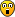Mathimagics
2017 Supporter

Posts: 1901
Joined: 27 May 2015
Location: Canberra

### Re: The hardest sudokus (new thread)

Mathimagics wrote:What, no steak knives? Unbelievable !!!steak knives? who do you think we are? this is a sudoku store, not Toys-'R'-us!
999_Springs

Posts: 585
Joined: 27 January 2007
Location: In the toilet, flushing down springs, one by one.

### Re: The hardest sudokus (new thread)

999_Springs wrote:
Pupp wrote:Just curious, but does anybody know what the hardest puzzles you can get with 32 clue symmetrical puzzles.

I was reading about the history of Sudoku, and when it first became popular in Japan, they arbitrarily decided that 32 clue symmetrical puzzles was best. The symmetry seems to be a cultural thing, but the 32 clues was decided arbitrarily, in order to keep the puzzles from being too hard.

interesting, do you have a source for them deciding that 32 clue puzzles were best?

i phoned up the sudoku store in puzzle-land and asked their high-clue hard puzzles department for 32 clue symmetric ones, and they said, well we have this wonderfully delightful non-minimal 37 clue puzzle by Mauricio, which is in perfectly mint condition and comes in a solid oak frame with a classy mahogany finish and galvanised rust-proof handles, available in small size for £9.99, medium for £14.99 or large for £19.99, and the store would be happy to remove 5 of the extra clues for you to satisfy your 32 clue requirement at only an extra £2.99 per puzzle. hurry, this one is almost out of stock!
Code: Select all
`6..|..2|..9.2.|.4.|.1...3|5..|2..---+---+---3..|194|5...1.|6.8|.3...5|273|..1---+---+---..4|..5|1...3.|.2.|.4.7..|4..|..8Mauricio's 37-clue with 5 clues removed, SE 11.2`

if this exquisite piece of artwork is too expensive for your liking, we also have in stock this beautiful relic of a patterns game puzzle also by Mauricio, 36 clues non-minimal fully symmetric, from patterns game 79; it comes in a sturdy balsa wood frame with a plastic exterior and foldable handles and is available in small size for £3.99, medium for £5.99 or large for £7.99. we can remove 4 extra clues to get to 32 clues at an additional £1.99 per puzzle. we can deliver these puzzles to your address in 3-5 working days for free, or an extra £4.99 for next day delivery
Code: Select all
`6..|1.2|.353..|...|4...51|..4|6..---+---+---7.5|2.8|..6...|...|...4..|7.9|1.8---+---+---..4|6..|51...6|...|..313.|9.5|..436 clues with 4 clues removed, SE 10.2`

now the thing about both of these puzzles is that they are MUCH easier than their SE rating would suggest because the placement of the clues means you can immediately invoke a "trick" called gurth's symmetry theorem on both puzzles which makes them much easier and doable by human players, and although they're still not easy, they are nowhere near as hard as other SE 10+ puzzles

if you are looking for 32 clue puzzles that don't have gurth's symmetry theorem, i once looked through champagne's hardest list to what happens if you fill in all the singles at the start of the puzzles and there were in fact a few puzzles in the 11.3-11.4 range with 33-34 clues after you do the singles, but it seems very unlikely that any of them would be symmetric, and i didn't keep the puzzles on this laptop anyway so i can't have another look right now

maybe i should become a modern art salesman or something

EDIT: i get the impression you probably could make this ssxsssxs's 46-clue SE 10.2 into a symmetric puzzle with a high clue count by removing clues and rearranging the rows and columns, since the clue pattern looks so nice at the start, and there is no gurth's ST on that. but i'm not going to do it

I was looking at the history of Sudoku. When it first became popular in Japan, someone arbitrarily decided that there should be a minimum of 32 clues in order to make sure the puzzles weren't too hard. Plus it was many years before someone wrote software to create puzzles with a press of a button. I'd think before software, in the early days anyway, Sudoku puzzles were created retro-actively by starting will a filled out board, and working backwards to create a puzzle.
Pupp

Posts: 246
Joined: 18 October 2019

### Re: The hardest sudokus (new thread)

999_Springs wrote:EDIT: i get the impression you probably could make this ssxsssxs's 46-clue SE 10.2 into a symmetric puzzle with a high clue count by removing clues and rearranging the rows and columns, since the clue pattern looks so nice at the start, and there is no gurth's ST on that. but i'm not going to do it

It has some 23 clue minimals, I wonder if there are many harder puzzles you can cut in half like that.
mith

Posts: 859
Joined: 14 July 2020

### Re: The hardest sudokus (new thread)

Updated the spreadsheet again. I finally started running the scripts on 24C, and did find a new 11.6 there. Compared with my previous post, there are five more 11.6s (two 21C, a 22C, a 23C, and the 24C) and one new 11.7 (23C). Quite a few more highly rated by q1, because I spent a few hours just running that on puzzles (and am now rating all the newly generated ones by q1 if they pass the q2 and skfr filters).
mith

Posts: 859
Joined: 14 July 2020

### Re: The hardest sudokus (new thread)

Mid-month update; I haven't updated the individual tabs (in fact, I may do away with these entirely in favor of a CSV), but I've added a new sheet for listing every puzzle meeting one of the following criteria: ER>=11.6 or tech_ER=D*FC+DFC or q1>=99000 or q2>=99100. Here they are in text form:

Code: Select all
`21C........1.....2.3...4.5.6.7...2.8....7..6...59.........16...4...5..1.7..8..3.....  ED=11.7/11.7/2.6  (DCFC+DFC) q1=95705 q2=97905........1....23.....45...6......1....5.7...8.9...5...3.6.8..7...75....4.2.......9  ED=11.7/1.2/1.2   (DCFC+DFC) q1=13293 q2=95102........1.......2...3..45......1..6....7.38...57..8....458..3...7.......6...9...2  ED=11.6/11.6/2.6  (DCFC+DFC) q1=95076 q2=98167........1.....2..3...45..6...1...2...4..7..8.86..4......3..9...48.5...7.5........  ED=11.6/1.2/1.2   (DCFC+DFC) q1=95031 q2=98005........1.....2..3...45..6...1...2...6..4..7.47..8......3..9...5........74.5...8.  ED=11.6/1.2/1.2   (DCFC+DFC) q1=95005 q2=97884........1.....2..3...45..6...1...2...6..4..7.74..8......3..9...47.5...8.5........  ED=11.6/1.2/1.2   (DCFC+DFC) q1=95005 q2=97884........1.......23..1..24......5.6....2..7.4.68.9.......3..1..4.5....7..9...8....  ED=10.3/10.3/9.2  (DLFC+FC)  q1=99319 q2=98854........1.....234...1.5........6...7...8...3..4...98...8.2...9.1........5.6.7...4  ED=11.2/11.2/9.2  (DCFC+MFC) q1=99244 q2=98766.................1..2.34.5...36.7....2.8.....5...2..7...5.......3..52.4.7.4...9..  ED=10.9/1.2/1.2   (DLFC+MFC) q1=99218 q2=98850........1.....2.3...4.5.6.7...2.8....7..6.1..9.........16.....4.5..1.7..8..3.....  ED=11.4/1.2/1.2   (DCFC+MFC) q1=99131 q2=98848........1.....2.3...3.4.5.....6.......1.7...4.8...97....5.1..7..6...8...9..2..4..  ED=10.8/1.2/1.2   (DCFC+FC)  q1=99081 q2=98868........1.....2..3..4.5..6...5....7..3....8..7..94......65...9..1...8....2.67....  ED=10.6/10.5/2.6  (DCFC+FC)  q1=99071 q2=98769........1.......23..1..24......5.6....2.67.4..8.9.......3..1..4.5....7..9...8....  ED=10.5/10.5/9.8  (DCFC+FC)  q1=99032 q2=98958........1..2..3.4..4..5.6.......4.7...41....38...9......17....2.5..6....9.....8..  ED=11.5/11.5/10.0 (DCFC+MFC) q1=97523 q2=99400........1.......2...3..45......1...6..5..73...4...8....5.8..7...87..3...6...2...9  ED=9.9/1.2/1.2    (DCFC+)    q1=96377 q2=9920522C........1.....2.3...4.5.6...3...1...4.78.....6..54.....4....7...9.....127...6.8..  ED=11.8/1.5/1.5   (DCFC+DFC) q1=98819 q2=97704........1..2..3.4..4..5.6.......7.8...43......1..9.5...9......63.78.....4....2.7.  ED=11.7/11.7/10.7 (DCFC+DFC) q1=95075 q2=97539........1.....2.3...4.5.6.....2...7..7...4..28...9.5....5..69...1.3.....6.8.4....  ED=11.7/11.7/9.4  (DCFC+DFC) q1=97299 q2=97638........1.....2.34..3.5.6.....6..7...7..8....9....1.4..8.5.....3.1....9.4....3..2  ED=11.7/11.7/8.0  (DLFC+DFC) q1=95031 q2=97177.....1..2....3..4....5..1.6..1..72...8......59......8...6..5...43..9....89.3.....  ED=11.7/11.7/2.6  (DCFC+DFC) q1=95459 q2=98416........1.....1..2...34..5...36......574.....8....2....46....7..7.5...6.9...6.1..  ED=11.7/11.7/2.6  (DCFC+DFC) q1=95012 q2=95198........1.....2.....3.4.56....7.......4.......5..3.42..26.....8.35..9...4...2.35.  ED=11.7/1.2/1.2   (DLFC+DFC) q1=95807 q2=98760........1....23.4.....452....1.....6.3...6.2.6.7...8....8.5.....5...4...1..6....9  ED=11.7/1.2/1.2   (DRFC+DFC) q1=75392 q2=95978........1.....2.3.....4.5.6..6.5...4.51...7..8..2......6.8...9..7..1.6..9....3...  ED=11.6/11.6/8.0  (DCFC+DFC) q1=95049 q2=98089........1.....2.3...4.5.6.......1......3...27.6..8.9...498..5...86.4....7....9...  ED=11.6/11.6/2.6  (DCFC+DFC) q1=98817 q2=97556........1....23.4.....452....1.3.....3...2.6.7..6....8..7.....9.5....4..8.9....1.  ED=11.6/11.6/2.6  (DLFC+DFC) q1=69294 q2=95511........1.....2.3.....3.42...15....6.6.1.....7...8.2...5.9.....4..2..78.8...4....  ED=11.6/1.2/1.2   (DCFC+DFC) q1=17776 q2=95085........1.....2.....3.4..5........6...7...4.3.6..3.7.5.35.6..7.8...5..4.9..8.....  ED=11.4/1.2/1.2   (DLFC+DFC) q1=95813 q2=98927..............1.23..4.5.6.......2.7...8.4.5...7.3....1..79....5.6..7....84....7..  ED=10.1/1.2/1.2   (DCFC+)    q1=99412 q2=99403........1.....2.34..1.3.5....3.6..5..6.7..2..8....9.....4.2...5.7....6..9..8.....  ED=10.5/10.5/10.4 (DCFC+FC)  q1=99384 q2=99378........1..2..3.4..4..5.6.....6.......7..8.2..9..1...5..98..4...7.....9.32...9...  ED=10.4/10.4/9.6  (DRFC+FC)  q1=99381 q2=99375........1.....2.34..4.3.5.....6..2....1.4...5.7...8....8....9...93.2..5.6....7...  ED=10.3/10.3/9.8  (DLFC+FC)  q1=99325 q2=99217...........1..2..3.4..5.67.....6.4....8..1..24..7...5....9...2.18......43....4...  ED=10.0/1.2/1.2   (DCFC+)    q1=99375 q2=98985.................1..2.34.5....6.......37.2..8.5..8..2..24...9...85......3...58.4.  ED=10.9/1.2/1.2   (DLFC+MFC) q1=99231 q2=98889.................1..2.34.5....6.......37.25...8..5..2..24...9...58......3...85.4.  ED=10.9/1.2/1.2   (DLFC+MFC) q1=99231 q2=98889.................1..2.34.5...36.2.....57......8..5..2..24...9...58......3...85.4.  ED=10.9/1.2/1.2   (DLFC+MFC) q1=99230 q2=98889.................1..2.34.5...36.2.....78......5..7..2..24...9...75......3...57.4.  ED=10.9/1.2/1.2   (DLFC+MFC) q1=99230 q2=98889.................1..2.34.5....6.......37.8..2.5..2..8..25.......84...9..3...52.4.  ED=10.9/1.2/1.2   (DLFC+MFC) q1=99218 q2=98850..............1.23..4.5.6.7.....2.7...8.4.5...7.3....1..79....5.6..7....84.......  ED=10.0/1.2/1.2   (DCFC+)    q1=99196 q2=99011.................1..2.34.5...36.......47.5....5..4..8..28.......45...9..3...28.4.  ED=10.9/1.2/1.2   (DLFC+MFC) q1=99182 q2=98911........1.....2.3...4.5.6.......3.2...7.4.5...4.1....8..8..9.5.47....8..6...8....  ED=10.2/1.2/1.2   (DCFC+FC)  q1=99165 q2=98915........1.....2.34.45.1.6.......75...73.5..1.8...........7...6..16.4...59........  ED=11.0/1.2/1.2   (DCFC+MFC) q1=99100 q2=98826........1.....2....13.4..5........46...7...35.46.3.1...6..5...48........9.5..6...  ED=10.9/1.2/1.2   (DLFC+MFC) q1=99091 q2=98879........1.....2....13.4..5........64...7...35.64.3.1...4..5...68........9.54.....  ED=10.9/1.2/1.2   (DLFC+MFC) q1=99091 q2=98879........1.....2.....3.4..5........6...46..7.3.7..3.4.5..7.6..4.3...5..7.89.......  ED=10.9/1.2/1.2   (DLFC+MFC) q1=99090 q2=98879........1.....2.....3.4..5........6...76..4.3.4..3.7.5..4.6..7.3...5..4.89.......  ED=10.9/1.2/1.2   (DLFC+MFC) q1=99090 q2=98879........1.....2.....3.4..5........6...76..4.3.4..3.7.5..4.6..7..3..5..4.89.......  ED=10.9/1.2/1.2   (DLFC+MFC) q1=99056 q2=98761.................1..2.34.5...36.......47.5....5..4..8..28.......45...9..3...82.4.  ED=11.0/1.2/1.2   (DCFC+MFC) q1=99042 q2=98758.................1..2.34.5...36.......78....4.5..4..7..25.......74.....93...52.4.  ED=10.7/1.2/1.2   (DCFC+FC)  q1=99032 q2=98418.................1..2.34.5...36.......57.2....8..5..2..24...9...58......3...85.4.  ED=10.9/1.2/1.2   (DLFC+MFC) q1=99024 q2=98774.................1..2.34.5...36.......475.....5...3.8..28.......35...9..4...28.3.  ED=11.0/1.2/1.2   (DLFC+MFC) q1=99009 q2=98538.................1..2.34.5.....25.4..2.3......65...3.7..386.....5...3.6.4..9.....  ED=10.7/1.2/1.2   (DCFC+FC)  q1=99006 q2=98766........1.....234...3.1.25...1..3..5.6..7....8..9.......2..1..4.7.....8.9..6.....  ED=10.9/1.2/1.2   (DCFC+MFC) q1=97520 q2=99406........1..2..3..4.5..6..2...5..7....8.1....32...8..9...8.2..6..6.7.....9.....4..  ED=10.8/1.2/1.2   (DCFC+FC)  q1=97592 q2=99393........1.....2.3...4.5.6....7..1..2.4..6.7..5....3.8..7........968.....4...7.9..  ED=10.9/1.2/1.2   (DCFC+MFC) q1=96252 q2=99376........1....23.4...56..7.......4..3..65..8...2..1......9.....758....9..6..9...5.  ED=10.6/1.2/1.2   (DCFC+FC)  q1=96138 q2=99348........1.....234...3.1.25...1..3..2.6....7..8..9.......4..1..5.9..6....7..8.....  ED=10.9/1.2/1.2   (DCFC+MFC) q1=97550 q2=99316........1.......23..1..34....2..5.4..4..6....7..8..9....5..1....8.4..5..6.47.....  ED=10.5/1.2/1.2   (DCFC+FC)  q1=96210 q2=99308........1.......23..1..34....2..5.4..4..6....7..8..9....5..1..4.8.4..5..6..7.....  ED=10.5/1.2/1.2   (DCFC+FC)  q1=96210 q2=99308........1.....2.34..4.3.5....2.6...5.7....6..8....92....3.1..5..9.8.....6....7...  ED=10.2/10.2/10.2 (DCFC+FC)  q1=97564 q2=99259........1..2..3....3..4..5...1.6.....5...4.6.7...5.8...6.4...9.2...9....8....67..  ED=10.8/1.2/1.2   (DCFC+FC)  q1=96511 q2=99172.....1..2..1....3..4..5.6.......3..7.6..8.9..7..2.......5.9..8..8.4.....9.6...4..  ED=10.5/1.2/1.2   (DRFC+FC)  q1=97574 q2=99164........1.....2.34..1.5.6....3.4..6..7...8....9.2.......4.2...6.8.7.....2....95..  ED=10.8/1.2/1.2   (DCFC+FC)  q1=97504 q2=99144........1..2..3.4..5..6.7.....38..6...62.....9.......4..9..8.3..7.6.....1...3.5..  ED=10.6/1.2/1.2   (DCFC+FC)  q1=96086 q2=99144........1.....234...3.1.25...1..5..3.6.....7.8..9.......5..1..4.9.8.....7...6....  ED=10.7/1.2/1.2   (DCFC+FC)  q1=95871 q2=9911023C................12..3.145.........4...16......5..371...35....8..749.....1...534..  ED=11.7/1.2/1.2   (DLFC+DFC) q1=95473 q2=98772........1.....2.3...4.5.6....754.....3...1...4.87......4.2..8...9.....128...6.7..  ED=11.6/11.6/2.6  (DCFC+DFC) q1=97576 q2=98765..............1..2.34.2..5....6..1...13.4...57......4..21.3.5...5...8.2.9.......3  ED=11.6/1.2/1.2   (DCFC+MFC) q1=97699 q2=97969........1..2..3....4.15..6......17...5.63..8.9.........3......84.5.8.6..6..4...3.  ED=11.6/1.2/1.2   (DCFC+MFC) q1=97510 q2=97586..............1..2.13.4..5...6..3....751...3.8...5.....47....1..5.7..9..6..4..5..  ED=11.6/1.2/1.2   (DCFC+MFC) q1=95846 q2=97793........1.....2.....3.4..5...6...4...3.....7.7...3.6.5.8.9......97.5..4.3.5.7..6.  ED=11.5/1.2/1.2   (DRFC+DFC) q1=95829 q2=99010........1.....2.....3.4..5.3......6...7...4...6..3.7.5.36.5..7.8..9.....9.5.6..4.  ED=11.5/1.2/1.2   (DRFC+DFC) q1=95829 q2=99010........1..1..2.3..3..4.5.....1..4...4..65...1.7...6.....8...5..1..53..69..2.....  ED=11.4/1.2/1.2   (DCFC+DFC) q1=95884 q2=98792........1.....2..3..4.3..5....6.73....5.8..9..73.4..8...8...5.9.4.8.....3...9....  ED=11.4/1.2/1.2   (DCFC+DFC) q1=95879 q2=98792........1.....2.3...4.1.5......2.6....6.571..89.........72......4.1.57...5..7..6.  ED=11.4/1.2/1.2   (DLFC+DFC) q1=95852 q2=96203........1.....2.3...4.1.5......2.6....6.571..89.........72....5.4.1..7...5..7..6.  ED=11.4/1.2/1.2   (DLFC+DFC) q1=95852 q2=96203........1.....2.....3.4..5........6...7...4.3.6..3.7.5.36.5..7.8..9.....9.5.6..4.  ED=11.4/1.2/1.2   (DLFC+DFC) q1=95801 q2=98848........1.....2.3..13.4.5........1.4...6..7...74.1..5.....3...58.54.....9.6...4..  ED=11.4/1.2/1.2   (DLFC+DFC) q1=95008 q2=95547..............1.23.12.4.5......6..7....52.....56..42.....4..1..84.....5.97..5....  ED=11.4/1.2/1.2   (DLFC+DFC) q1=95004 q2=95542..............1.23.12.4.5......6..7....52.....56..42....54..1..84.......97..5....  ED=11.4/1.2/1.2   (DLFC+DFC) q1=95003 q2=95539..............1.23.12.4.5......6..7....52.....65..42....64..1..84.......97..5....  ED=11.4/1.2/1.2   (DLFC+DFC) q1=87953 q2=95484........1.....2..3..4.5.67.....6.48..4.5......689....5..5.......8..7..6.7....53..  ED=11.4/1.2/1.2   (DCFC+DFC) q1=29064 q2=95290........1.....2..3..4.5.67.....6.48..4.5......689....5..5.......8..7.56.7.....3..  ED=11.4/1.2/1.2   (DCFC+DFC) q1=29064 q2=95290........1..2..3.4..5..6.7.....4...3...6..1..55...8.2....5.7.9...7.9...5.98.......  ED=10.5/10.5/10.4 (DCFC+FC)  q1=99379 q2=99178........1..2..3....4..5..2......67...5..8..4.62..9..8...5.4.....8...9..42..8...9.  ED=11.0/1.2/1.2   (DLFC+MFC) q1=99105 q2=99356........1.......23..1..24....3..5....6..4.5..7.48.......5..1.4.4..6..9..8...74...  ED=10.5/1.2/1.2   (DCFC+FC)  q1=99099 q2=99108........1.......2...1.234.....5...6...6.34..2.7....3....2.46.1...48.....9....2..4  ED=10.7/1.2/1.2   (DCFC+FC)  q1=99077 q2=99142........1.......2...1.234.....5...6...6.34..2.7....34...2.46.1...48.....9....2...  ED=10.7/1.2/1.2   (DCFC+FC)  q1=99077 q2=99142........1.....2.34..3.1.5....1.2..5..6....7..8.5..9.....2.4...5.9...8...5..6..2..  ED=10.5/1.2/1.2   (DCFC+FC)  q1=99146 q2=98921........1.....2.....3.4..56..4...1...3...7..25...6..38..69......4..5..838.5......  ED=11.1/1.2/1.2   (DCFC+MFC) q1=99143 q2=99003........1.....2.....3.4..56..4...1...3...7..25...6..83..69......4..5..388.5......  ED=11.1/1.2/1.2   (DCFC+MFC) q1=99143 q2=99003..............1.23..1.425...1...64..2.57.....4..8...5..5.....9..6.......1...652..  ED=11.2/1.2/1.2   (DCFC+MFC) q1=99122 q2=98836........1.....2..3.13.4..5.........4..56......74.3.5....1.7...58.....34.9....3.7.  ED=11.2/1.2/1.2   (DCFC+MFC) q1=99122 q2=98836.................1..2.34.5...36.......475...3.5.....8..25...9...384.....4...28.3.  ED=10.9/1.2/1.2   (DLFC+MFC) q1=99115 q2=98783.................1..2.34.5.....26.3..25...74..364.......38.......495...3.5.....6.  ED=10.9/1.2/1.2   (DLFC+MFC) q1=99114 q2=98782........1.....2.....3.4.56...5...3.7.3..5..8.6..4......549....6.8.......3...6.84.  ED=10.6/1.2/1.2   (DCFC+FC)  q1=99111 q2=98775........1.....2.....3.4.56...5...3.7.3..5..846..4......549......8.......3...6.84.  ED=10.6/1.2/1.2   (DCFC+FC)  q1=99111 q2=98775........1.....2.....3.4.56...5...3.7.3..5..846..4......549......8...4...3...6.8..  ED=10.6/1.2/1.2   (DCFC+FC)  q1=99111 q2=98775........1.....2.....3.4.56...5...3.7.3..5.68.6..4......549......8.......3...6.84.  ED=10.6/1.2/1.2   (DCFC+FC)  q1=99111 q2=98775........1.....234...3.1.25.....6......2..4..3.6.....7...5..1..22.6..3..48..9.....  ED=10.9/1.2/1.2   (DDFC+MFC) q1=99102 q2=98826........1.....2.34.45.1.6.......75...73.5..1.8.........16.4....5..7...6.9....5...  ED=11.0/1.2/1.2   (DCFC+MFC) q1=99100 q2=98826........1.....234...3.5.......2......2..6.75.5.87.4.....5.....9.7....26.3....6..5  ED=10.9/1.2/1.2   (DDFC+MFC) q1=99099 q2=98825........1.....234...3.5.......2.5....2..6.7..5.87.4.....5.....9.7....26.3....6..5  ED=10.9/1.2/1.2   (DDFC+MFC) q1=99099 q2=98825........1....23.45..51..2....13...2..6...27..8....9.....34......9..6.3..2....8...  ED=10.4/1.2/1.2   (DCFC+FC)  q1=99077 q2=98813........1.....2.34..1.4.2....3.5..2..6....7..8....9.....7.3...2.3...85..9..62....  ED=10.3/10.3/10.1 (DCFC+FC)  q1=99066 q2=98796........1.....2.34..1.4.5....2.3...5.5...6...7..8..2....4.2..5..6...7...8..4..9..  ED=10.6/1.2/1.2   (DCFC+FC)  q1=99065 q2=98937........1.....2.34..1.4.5....2.3...5.5...6...7..8..2....4.2..5..65..7...8.....9..  ED=10.5/1.2/1.2   (DRFC+FC)  q1=99065 q2=98850........1.....2.34..4.1.5....1.2..5..6...57..8....9.....2.3...5.9.6..2..5....8...  ED=10.5/1.2/1.2   (DCFC+FC)  q1=99058 q2=98847........1..2..3.4..3..5.6......6......7.82.3..8.1....5..89....3.4...8...72.....8.  ED=10.5/1.2/1.2   (DDFC+FC)  q1=99039 q2=99067..............1..2.13.4..5....46.....54..3.6..6..5.7....56...1.8...1.3..9...3....  ED=11.3/1.2/1.2   (DCFC+MFC) q1=99025 q2=98795........1.....2....34.5..6.......6....17..45..65.1...3.5..4.1..8......3.9..3...4.  ED=10.4/1.2/1.2   (DLFC+FC)  q1=99020 q2=98505........1.....2.....1.3..45.1..5..633........6..7......4.3..8...534..9..1...6...4  ED=10.7/1.2/1.2   (DCFC+FC)  q1=97641 q2=99398........1.....2.3...4.5.6.7..78...5..6..7....94....7....9.6.8...7...3..24..1.....  ED=10.3/10.3/9.8  (DCFC+FC)  q1=97360 q2=99378........1.....2.....3.4..5...4...6...3.4.5.7.7...3.....8.9......95.7..4.3.7.5..6.  ED=10.6/10.6/3.4  (DCFC+FC)  q1=96598 q2=99328........1.....2.....3.4..5...4...6...3.4.5.7.7...3.....8.9......97.5..4.3.5.7..6.  ED=10.6/10.6/3.4  (DCFC+FC)  q1=96598 q2=99328........1.....2.3..34.1.5.....6..4...7.....8..81.4...5.48.3..5..5...98..7........  ED=11.0/1.2/1.2   (DLFC+MFC) q1=97959 q2=99315........1....23....245...3....6..7...56.3..4.2..4......32..6.5..6..5...89........  ED=10.7/1.2/1.2   (DCFC+FC)  q1=96074 q2=99262........1.....2.....3.4..5...4...6...3.4...7.7...3.5...8.9......95.7..4.3.7.5..6.  ED=11.3/1.2/1.2   (DCFC+MFC) q1=95821 q2=99261...........1..2..3.3..4.25.......6.7...48.....2...384...3.5.....5.2...8.9...3...6  ED=11.1/1.2/1.2   (DCFC+MFC) q1=95708 q2=99230........1.....2.....3.4.56...5.....4.3.....784...3.95...64.7....5..6..3.9.4......  ED=10.6/1.2/1.2   (DCFC+FC)  q1=95568 q2=99206..............1..2..3.4..5........6...4...7.3.6..3.4.5.37.6..4.2.6.5..3.8..9.....  ED=10.6/1.2/1.2   (DCFC+FC)  q1=95571 q2=99205........1.....2..3..4.5..6....5.......5...7.4.1..7.6...56.1..4.8...6..7.9..8..1..  ED=10.8/1.2/1.2   (DCFC+FC)  q1=97025 q2=99187........1.....2.3...3.4.56..3..5.67.5........6....8....4.9..3...7.4.....3...6.74.  ED=10.5/10.5/2.6  (DLFC+FC)  q1=97048 q2=99177..............1..2..3.4..56..4.......3.7..2..6...3..85..5..98...4..8..638.6......  ED=10.6/1.2/1.2   (DCFC+FC)  q1=97556 q2=99175........1.....2..3..4.5..6...5...7...4.8..3..7...4..16..6..9....5..1..741.7......  ED=10.6/1.2/1.2   (DCFC+FC)  q1=97556 q2=99175........1.....2..3..4.5..67..5.......4.8..3..7...4..16..6..9....5..1..741.7......  ED=10.6/1.2/1.2   (DCFC+FC)  q1=97556 q2=99175........1.....2.....3.4.56...473..5...5.6..8..9....7....64......3..8....85...6.4.  ED=11.4/1.2/1.2   (DCFC+MFC) q1=97097 q2=99146........1.....2.3..34.1.5.....6...7...8....5..71.5...3.45.7..1.7..9..4..8........  ED=10.8/1.5/1.5   (DCFC+FC)  q1=97264 q2=99138..............1..2..3.4.56....7...8...5.9.3.6.89.3.4....6..4....5.9.....93..6....  ED=10.9/1.2/1.2   (DLFC+MFC) q1=97717 q2=99122.......12......3.4.....5.6...3.4..2..4.7..6..86...9.....4.1..3..9.6.....7....8..6  ED=11.3/1.2/1.2   (DCFC+MFC) q1=97346 q2=99106.......12......3.4.....5.6...3.4..2..4.7..6..86...9....9.6.....6.4.1..3.7....8...  ED=11.3/1.2/1.2   (DCFC+MFC) q1=97346 q2=99106........1.....2.34..3.1.25...1.4.....6...7...42.8.......4.5.....82..69..7..2.....  ED=11.3/1.2/1.2   (DCFC+MFC) q1=97355 q2=99101...........1..2..3.2..4..5....4.6.7..6.2....57...5.....5..7..68.7..2..4.9..8.....  ED=10.8/1.2/1.2   (DCFC+FC)  q1=96081 q2=9910124C........1.....2.3...4.5.6.....7....8..5.6.1...81.9.4....6......41...69..59..4...6  ED=11.6/11.6/9.0  (DLFC+DFC) q1=95049 q2=97556........1..2..3..4.5..6.27.....8.52.5..9.6...8.......6..76..4...6.....1.2...7.8..  ED=11.7/1.2/1.2   (DCFC+MFC) q1=95455 q2=98132........1.....2..3..4.3..5....6.73...63.4..8.7.5.8..9...8...5.9.3..9....4....8...  ED=11.6/1.2/1.2   (DCFC+MFC) q1=95465 q2=98149........1.....2..3..4.3..5....6.73...65.8..9.7.3.4..8...8...5.9.4...8...3...9....  ED=11.5/1.2/1.2   (DCFC+DFC) q1=89756 q2=96626........1..2..3..4.5..6.27.....8.52.5..9.6...8.......6..76..1...6.....4.2...7.8..  ED=11.5/1.2/1.2   (DCFC+DFC) q1=87769 q2=96528........1.......2...1.234.....5...6...6.34..2.7.2..3....2.46.1...48..2..9.......4  ED=10.7/1.2/1.2   (DCFC+FC)  q1=99077 q2=99142........1.......2...1.234.....5...6...6.34..2.7.2..34...2.46.1...48..2..9........  ED=10.7/1.2/1.2   (DCFC+FC)  q1=99077 q2=99142........1....12.3..134..5......6.4...453...7.8.........517....4.6..5....9.....75.  ED=10.8/1.2/1.2   (DCFC+FC)  q1=99131 q2=98875........1....23.45.251..6.......72...47....1.8.2..........7..6..615....29....2...  ED=11.0/1.2/1.2   (DCFC+MFC) q1=99100 q2=98826........1..2..3....3..4.25.........6.7.3..48.2...8...3...43.....4...87..3.975....  ED=11.0/1.2/1.2   (DCFC+MFC) q1=99099 q2=98825........1.....2.....3.4.25......4..6..42..53..7..3.4....5..382...7.8....9..5....3  ED=10.6/1.2/1.2   (DCFC+FC)  q1=99014 q2=98812........1.....2.3...3.4.56......4..7..42......5..3.48..35..9....86......4...6.85.  ED=11.0/1.2/1.2   (DCFC+MFC) q1=99012 q2=98783.................1..2.34.5.....26.3..25...74..364.......38.......49.5..3.5..4..6.  ED=10.9/1.2/1.2   (DLFC+MFC) q1=99007 q2=98886.................1..2.34.5...36.......47.5..3.5..4..8..25...9...384.....4...28.3.  ED=10.9/1.2/1.2   (DLFC+MFC) q1=99007 q2=98886........1.....2.3...2.3.4.5......6...1..4..5.3.5..7....53.2..1.8..9.....92..5..4.  ED=10.6/1.2/1.2   (DDFC+FC)  q1=97577 q2=99377........1.....2.3...4.3.5....3..4....6..7..5..7.3..4....7.5.6..8....3..99.6.4.3..  ED=11.1/1.2/1.2   (DCFC+MFC) q1=95712 q2=99236........1....23.....45...2...6.5.....4.7..6..7....2.4...728..6..8....9..4.56...7.  ED=10.8/1.2/1.2   (DCFC+FC)  q1=97579 q2=99219........1.....2.....3.4.56........78..5...3.4.4..3.95..364.7....94......5...6..3.  ED=10.6/1.2/1.2   (DCFC+FC)  q1=95568 q2=99206..............1..2..3.4..56...7..2....4....3..6..3..85.35..98...86......4...8..63  ED=10.6/1.2/1.2   (DCFC+FC)  q1=97556 q2=99175........1.....2..3..4.5..6....7..3....5...84..8..4..16.18.......46..9...5...1..84  ED=10.6/1.2/1.2   (DCFC+FC)  q1=97556 q2=99175........1.....2..3..4.5..67...8..3....5....4..7..4..16.17.......46..9...5...1..74  ED=10.6/1.2/1.2   (DCFC+FC)  q1=97556 q2=99175........1.....2.....3.4..5......67....4.8..3.675.9..8...8..9....4..3...835.8...9.  ED=11.2/1.2/1.2   (DCFC+MFC) q1=97005 q2=99160........1.....2.....3.4..5...4.6..3..3...78..7.5.9..6...6..9....4..3...635.6...9.  ED=11.4/1.2/1.2   (DCFC+MFC) q1=97528 q2=99154........1....23.4..456..2.......2.1..32.6.4..7....4....53..61...6..5...89........  ED=10.9/10.9/10.7 (DLFC+MFC) q1=97302 q2=99102........1.....2.3...4.5.6.....7....8..9.6.4...75.4.92...1......65..1....94...61..  ED=11.3/1.2/1.2   (DCFC+MFC) q1=96417 q2=99102`

Particularly noteworthy among the new ones is the 24C 11.7, which again breaks the SE record for +MFC.

The actual "hardest" among these may be this one:

Code: Select all
`........1..2..3.4..4..5.6.......4.7...41....38...9......17....2.5..6....9.....8..  ED=11.5/11.5/10.0 (DCFC+MFC) q1=97523 q2=99400`
mith

Posts: 859
Joined: 14 July 2020

### Re: The hardest sudokus (new thread)

mith wrote:The actual "hardest" among these may be this one:
Code: Select all
`........1..2..3.4..4..5.6.......4.7...41....38...9......17....2.5..6....9.....8..  ED=11.5/11.5/10.0 (DCFC+MFC) q1=97523 q2=99400`

"Actual hardest" doesn't mean anything, as there's no unique rating. The interest of any rating depends on its purpose and on its adequation to this purpose.
As for this puzzle in particular, it is in B5B, which is relatively high for a SER 11.5, but it is still very far from the highest SER or from the hardest in the BpB classification.
Maybe I didn't understand what you meant by "among these".
denis_berthier
2010 Supporter

Posts: 3289
Joined: 19 June 2007
Location: Paris

PreviousNext